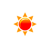### Percentage Difference Calculator

 Example: What is the percentage difference between 10 and 20? Anwser: ((20 - 10) ÷ ((20+10) ÷ 2) × 100 = 66.66% What is the percentage difference between and ? Answer: %

The Percentage Difference Calculator tool enables you to calculate the The difference between two numbers divided by the average of the two numbers. Then shown as a percentage.

#### The Percent Difference Formula

You can use the percent difference formula to find the percentage difference of two numbers:

Percentage Difference
=
New Number - Original Number / (New Number + Original Number) ÷ 2
× 100

Example: What is the percentage difference between 12 and 20 ?

Percentage Difference(%)
=
20 - 12 / (20 + 12) ÷ 2
× 100

Anwser: 50%

To know how to calculate percentage difference between two numbers? Please use our percentage difference calculator.

#### References

More references for Percentage Difference Calculation

#### Local Weather Status

Ashburn, United States
20th January, 2020 Monday
Clear Sky-2.78 - 1
Humidity: 46 %
Wind: 6.7 km/h